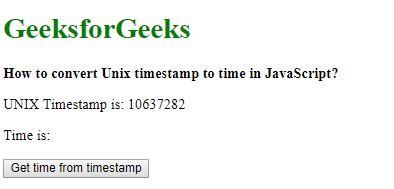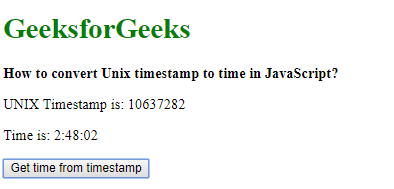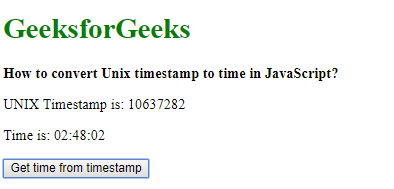# How to convert Unix timestamp to time in JavaScript?

UNIX timestamps can be converted to time using 2 approaches:

Method 1: Using the toUTCString() method:
As JavaScript works in milliseconds, it is necessary to convert the time into milliseconds by multiplying it by 1000 before converting it. This value is then given to the Date() function to create a new Date object.

The toUTCString() method is used to represent the Date object as a string the UTC time format. The time from this date string can be found by extracting from the 11th to last to the 4th to the last character of the string. This is extracted using the slice() function. This string is the time representation of the UNIX timestamp.

Syntax:

 `dateObj = ``new` `Date(unixTimestamp * 1000); ` `utcString = dateObj.toUTCString(); ` `time = utcString.slice(-11, -4); `

Example:

 ` ` `<``html``> ` ` `  `<``head``> ` `    ``<``title``> ` `      ``How to convert Unix timestamp ` `      ``to time in JavaScript? ` `  `` ` ` ` ` `  `<``body``> ` `    ``<``h1` `style``=``"color: green"``> ` `      ``GeeksforGeeks ` `  `` ` `    ``<``b``> ` `      ``How to convert Unix  ` `      ``timestamp to time in JavaScript? ` `  `` ` `    ``<``p``> ` `      ``UNIX Timestamp is: 10637282 ` `  `` ` `    ``<``p``>Time is: <``span` `class``=``"output"``> ` `      `` ` `  `` ` ` `  `    ``<``button` `onclick``=``"convertTimestamptoTime()"``> ` `      ``Get time from timestamp ` `  `` ` `    ``<``script` `type``=``"text/javascript"``> ` `        ``function convertTimestamptoTime() { ` `            ``unixTimestamp = 10637282; ` ` `  `            ``// convert to milliseconds and  ` `            ``// then create a new Date object ` `            ``dateObj = new Date(unixTimestamp * 1000); ` `            ``utcString = dateObj.toUTCString(); ` ` `  `            ``time = utcString.slice(-11, -4); ` ` `  `            ``document.querySelector( ` `              ``'.output').textContent = time; ` `        ``} ` `    `` ` ` ` ` `  ` `

Output:

• Before clicking the button:• After clicking the button:Method 2: Getting individual hours, minutes and seconds
As JavaScript works in milliseconds, it is necessary to convert the time into milliseconds by multiplying it by 1000 before converting it. This value is then given to the Date() function to create a new Date object.

Each part of the time is extracted from the Date object. The hour’s value in UTC is extracted from the date using the getUTCHours() method. The minute’s value in UTC is extracted from the date using the getUTCMinutes() method. The second’s value in UTC is extracted from the date using the getUTCSeconds() method.

The final formatted date is created by converting each of these values to a string using the toString() method and then padding them with an extra ‘0’, if the value is a single-digit by using the padStart() method. The individual parts are then joined together with a colon(:) as the separator. This string is the time representation of the UNIX timestamp.

Syntax:

 `dateObj = ``new` `Date(unixTimestamp * 1000); `   `// Get hours from the timestamp ` `hours = dateObj.getUTCHours(); `   `// Get minutes part from the timestamp ` `minutes = dateObj.getUTCMinutes(); `   `// Get seconds part from the timestamp ` `seconds = dateObj.getUTCSeconds(); `   `formattedTime = hours.toString().padStart(2, ``'0'``) + ``':'` `+  ` `                ``minutes.toString().padStart(2, ``'0'``) + ``':'` `+  ` `                ``seconds.toString().padStart(2, ``'0'``); `

Example:

 ` ` `<``html``> ` ` `  `<``head``> ` `    ``<``title``> ` `      ``How to convert Unix timestamp  ` `      ``to time in JavaScript? ` `  `` ` ` ` ` `  `<``body``> ` `    ``<``h1` `style``=``"color: green"``> ` `      ``GeeksforGeeks ` `  `` ` `    ``<``b``> ` `      ``How to convert Unix timestamp ` `      ``to time in JavaScript? ` `  `` ` `    ``<``p``>UNIX Timestamp is: 10637282 ` `    ``<``p``>Time is: <``span` `class``=``"output"``> ` `      `` ` `  `` ` ` `  `    ``<``button` `onclick``=``"convertTimestamptoTime()"``> ` `      ``Get time from timestamp ` `  `` ` `    ``<``script` `type``=``"text/javascript"``> ` `        ``function convertTimestamptoTime() { ` `            ``unixTimestamp = 10637282; ` ` `  `            ``// convert to milliseconds ` `            ``// and then create a new Date object ` `            ``dateObj = new Date(unixTimestamp * 1000); ` ` `  `            ``// Get hours from the timestamp ` `            ``hours = dateObj.getUTCHours(); ` ` `  `            ``// Get minutes part from the timestamp ` `            ``minutes = dateObj.getUTCMinutes(); ` ` `  `            ``// Get seconds part from the timestamp ` `            ``seconds = dateObj.getUTCSeconds(); ` ` `  `            ``formattedTime = hours.toString().padStart(2, '0') + ':' + ` `                ``minutes.toString().padStart(2, '0') + ':' + ` `                ``seconds.toString().padStart(2, '0'); ` ` `  `            ``document.querySelector('.output').textContent = formattedTime; ` `        ``} ` `    `` ` ` ` ` `  ` `

Output:

• Before clicking the button:• After clicking the button:My Personal Notes arrow_drop_upIm listening

If you like GeeksforGeeks and would like to contribute, you can also write an article using contribute.geeksforgeeks.org or mail your article to contribute@geeksforgeeks.org. See your article appearing on the GeeksforGeeks main page and help other Geeks.

Please Improve this article if you find anything incorrect by clicking on the "Improve Article" button below.

Article Tags :

Be the First to upvote.

Please write to us at contribute@geeksforgeeks.org to report any issue with the above content.